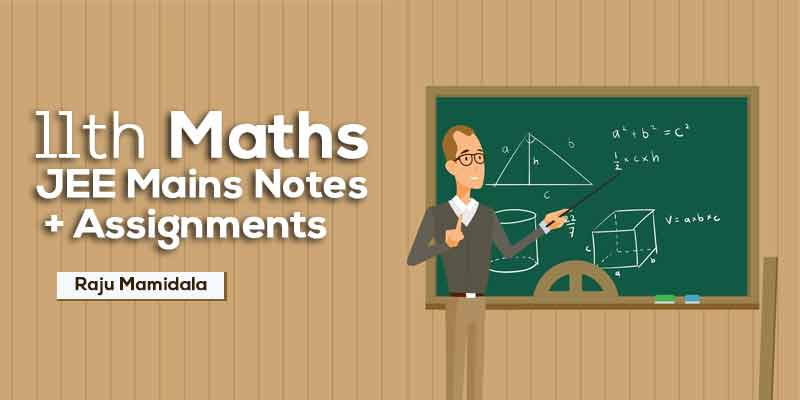## by Raju Mamidala

4.7 (112 Users)
JEE Mains , Maths
Board: CBSE & State Boards , Language: English

Mode- Online
Study Notes- 38
Validity- Till 31 Mar 2020
Language- English
No. of Views- Unlimited

## WHAT WILL I LEARN?

We aim to prepare this class students with a complete study material so that the students can score better in the Mains exam.

Simple and easy to understand.

Understand the level of IIT & JEE concepts by these quick revision notes.

Students can test themselves with assignments which are provided here.

## Curriculum

Compound angles

Continuity

Functions

Limits

• Limits - Class Notes
• Limits - Work Assignment

mathematical induction

• mathematical induction - Class Notes
• mathematical induction - Work Assignment

change of axis

• change of axis - Class Notes & Work Assignment

complex numbers

• complex numbers - Class Notes
• complex numbers - Work Assignment

DEMOIVRE'S THEOREM

• DEMOIVRE'S THEOREM - Class Notes & Work Assignment

dot product of two vectors

• dot product of two vectors - Class Notes & Work Assignment

errors

• errors - Class Notes
• errors - Work Assignment

heights and distances

• heights and distances - Class Notes
• heights and distances - Work Assignment

hyperbolic functions

• hyperbolic functions - Class Notes
• hyperbolic functions - Work Assignment

Locus

• Locus - Class Notes
• Locus - Work Assignment

maxima and minima

• maxima and minima - Class Notes
• maxima and minima - Work Assignment

Partial differentiation

• Partial differentiation - Class Notes
• Partial differentiation - Work Assignment

Periodicity

• Periodicity - Class Notes & Work Assignment

properties of triangles

• properties of triangles - Class Notes & Work Assignment

Rate of change

• Rate of change - Class Notes
• Rate of change - Work Assignment

Straight lines

• Straight lines - Class Notes
• Straight lines - Work Assignment

TANGENT AND NORMALS

• TANGENT AND NORMALS - Class Notes & Work Assignment

transformations

• transformations - Class Notes
• transformations - Work Assignment

Trigonometric Ratios

• Trigonometric Ratios - Class Notes
• Trigonometric Ratios - Work Assignment### Raju Mamidala

Mathematics | Class 6th to Competitive exams | 15+ Years Experience | Working in Geethanjali International School in Hyderabad from past 5 years. He Created an Educational Channel for improvement in various aspects for students in IIT Foundation.

## Student's Feedback## 600 399

Includes 5 % GST

Mode- Online

Study Notes- 38

Validity- Till 31 Mar 2020

Language- English

No. of Views- Unlimited